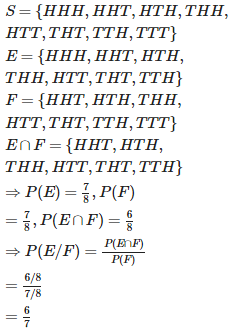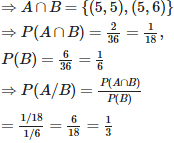# Probability and Statistics - Online Test

Q1. A batsman scores runs in 10 innings as 38,70,48,34,42,55,63,46,54 and 44 , then the mean score is
Explaination / Solution:

Mean (x) =

= 494/10

= 49.4

Q2. A coin is tossed three times, if E : head on third toss , F : heads on first two tosses. Find P(E|F)
Explaination / Solution:Q3.
Direction: Study the given information carefully and answer the questions that follow:

A basket contains 5 red, 4 blue, 3 green stones.
If two stones are picked at random, what is the probability that both are blue?
Explaination / Solution:

Probability if both is blue
4C2/12C2 = 6/66 → 1/11

Q4. The S.D. of the observations 22,26,28,20,24,30 is
Explaination / Solution:

Q5. A coin is tossed three times, E : at most two tails , F : at least one tail. Find P(E|F)
Explaination / Solution:Q6.
Direction: Study the given information carefully and answer the questions that follow:

A basket contains 5 red, 4 blue, 3 green stones.
If four balls are picked at random, what is the probability that two are blue and two are red?
Explaination / Solution:

Probability if two are Blue and two are Red
[(4C2*5C2)/12C4] = (6*10)/495 → 4/33

Q7. If the angle between two lines of regression is  , then it represents
Explaination / Solution:

here

hence r = 0, therefore there is no linear correlation.

Q8. A black and a red dice are rolled. Find the conditional probability of obtaining a sum greater than 9, given that the black die resulted in a 5.
Explaination / Solution:

n(S)=36.
Let A = event of getting sum greater than 9.
= {(4,6),(5,5),(6,4),(5,6),(6,5),(6,6)}
And B = event of getting 5 on black die.
={(5,1),(5,2),(5,3),(5,4),(5,5),(5,6)}Q9.
Direction: Study the given information carefully and answer the questions that follow:

A basket contains 5 red, 4 blue, 3 green stones.
If three balls are picked at random, what is the probability that at least one is green?
Explaination / Solution:

Probability if at least one is Green
[1-(9C3/12C3)] = 84/220 → 34/55

Q10. The two lines of regression are 2x - 7y + 6 = 0 and 7x – 2y +1 = 0. What is correlation coefficient between x and y ?
Explaination / Solution:

but sign of $\rho$ is same as the sign of

therefore,